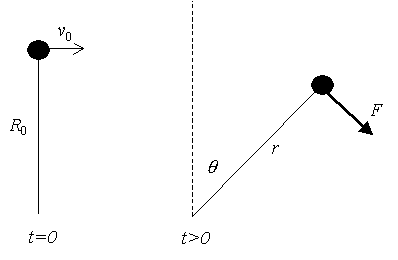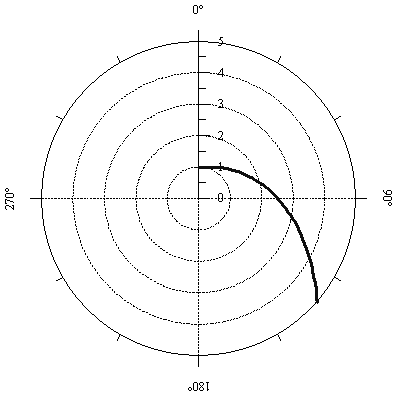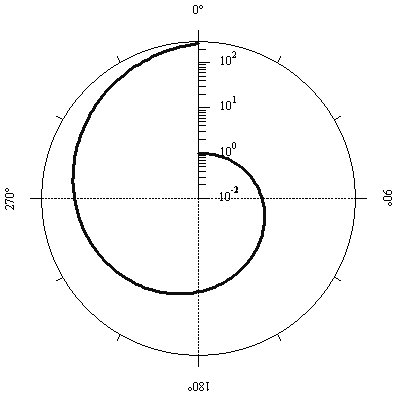At t=0 the angular velocity of the mass isand the string breaks.  For later times a force acts which keeps the angular velocityconstant at.  The force has no radial component, i.e.,.  Find.First, write Newton’s second law:(0.1)But,so(0.2)The first equation is easily solved:(0.3)and so(0.4)where a and b are constants to be determined.  Sinceand, it is easy to show that.  Therefore(0.5)Since,(0.6)Finally, from the equation (0.2) for F,. (0.7)Early trajectory of m for R0=1Trajectory of m for R0=1 over 3600 (note log scale for r)